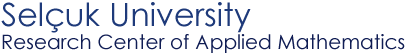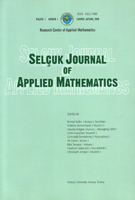Selçuk Journal of Applied Mathematics www.selcuk.edu.trSelçuk Journal of
Applied Mathematics

Winter-Spring, 2002
Volume  3
Number 1

Research Center of
Applied Mathematics

SJAM Winter-Spring 2002, Volume 3 - Number 1

Good solutions of fully nonlinear parabolic equations

Tran Duc Van1 , Tran Van Bang2

1 Hanoi Institute of Mathematics, P.O. Box 631 Bo Ho, 10.000 Hanoi, Vietnam;
email: tdvan@thevinh.ncst.ac.vn

2 Hanoi Pedagogical University, Xuan hoa, Hanoi, Vietnam;

Summary
In this paper, we introduce the notion of a "good"  solution of a fully nonlinear parabolic equation and show that "good" solutions are equivalent to Lp -viscosity solutions of such equations. The results here generalize the ones in  about "good" solutions of fully nonlinear elliptic equations. We give here an explicit construction of parabolic equations with Lp –strong solutions that approximate some nonlinear parabolic equation and its Lp -viscosity solution.

Key words

 SJAM Summer-Autumn 2002, Volume 3 - Number 1 Good solutions of fully nonlinear parabolic equations Tran Duc Van1 , Tran Van Bang2 1 Hanoi Institute of Mathematics, P.O. Box 631 Bo Ho, 10.000 Hanoi, Vietnam;    email: tdvan@thevinh.ncst.ac.vn 2 Hanoi Pedagogical University, Xuan hoa, Hanoi, Vietnam;    Received: April 02, 2002 Summary In this paper, we introduce the notion of a "good"  solution of a fully nonlinear parabolic equation and show that "good" solutions are equivalent to Lp -viscosity solutions of such equations. The results here generalize the ones in  about "good" solutions of fully nonlinear elliptic equations. We give here an explicit construction of parabolic equations with Lp –strong solutions that approximate some nonlinear parabolic equation and its Lp -viscosity solution. Key words Lp-viscosity solutions, good solutions, strong solutions, fully nonlinear parabolic equations

Mathematics Subject Classification (1991): 35J60, 35J65, 49L25

* This research was supported in part by National Council on  Natural Science, Vietnam.

Article in PS format (128 Kb)
 The article is available to read in  Ghostview format
 [Home] [contact]